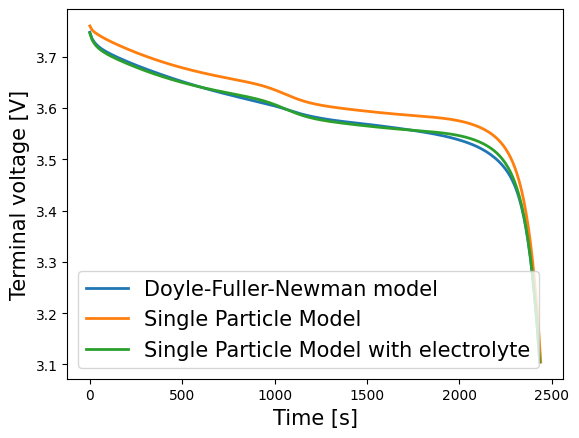Tip

An interactive online version of this notebook is available, which can be accessed via# Compare lithium-ion battery models#

We compare three one-dimensional lithium-ion battery models: the Doyle-Fuller-Newman (DFN) model, the single particle model (SPM), and the single particle model with electrolyte (SPMe). Further details on these models can be found in .

## Key steps:#

Comparing models consists of 6 easy steps:

2. Process parameters

3. Mesh the geometry

4. Discretise models

5. Solve models

6. Plot results

But, as always we first import pybamm and other required modules

:

%pip install "pybamm[plot,cite]" -q    # install PyBaMM if it is not installed
import pybamm
import os
os.chdir(pybamm.__path__+'/..')

import numpy as np
import matplotlib.pyplot as plt

WARNING: You are using pip version 22.0.4; however, version 22.3.1 is available.
You should consider upgrading via the '/home/mrobins/git/PyBaMM/env/bin/python -m pip install --upgrade pip' command.
Note: you may need to restart the kernel to use updated packages.


Since the three models we want to compare are already implemented in PyBaMM, they can be easy loaded using:

:

dfn = pybamm.lithium_ion.DFN()
spme = pybamm.lithium_ion.SPMe()
spm = pybamm.lithium_ion.SPM()


To allow us to perform the same operations on each model easily, we create a dictionary of these three models:

:

models = {"DFN": dfn, "SPM": spm, "SPMe": spme}


Each model can then be accessed using:

:

models["DFN"]

:

<pybamm.models.full_battery_models.lithium_ion.dfn.DFN at 0x7f917c709f40>


For each model, we must also provide a cell geometry. The geometry is different for different models; for example, the SPM has solves for a single particle in each electrode whereas the DFN solves for many particles. For simplicity, we use the default geometry associated with each model but note that this can be easily changed.

:

geometry = {"DFN": dfn.default_geometry, "SPM": spm.default_geometry, "SPMe": spme.default_geometry}


## 2. Process parameters#

For simplicity, we use the default parameters values associated with the DFN model, but change the current function to be an input so that we can quickly solve the model with different currents

:

param = dfn.default_parameter_values
param["Current function [A]"] = "[input]"


It is simple to change this to a different parameter set if desired.

We then process the parameters in each of the models and geometries using this parameter set:

:

for model_name in models.keys():
param.process_model(models[model_name])
param.process_geometry(geometry[model_name])


## 3. Mesh geometry#

We use the defaults mesh properties (the types of meshes and number of points to be used) for simplicity to generate a mesh of each model geometry. We store these meshes in a dictionary of similar structure to the geometry and models dictionaries:

:

mesh = {}
for model_name, model in models.items():
mesh[model_name] = pybamm.Mesh(geometry[model_name], model.default_submesh_types, model.default_var_pts)


## 4. Discretise model#

We now discretise each model using its associated mesh and the default spatial method associated with the model:

:

for model_name, model in models.items():
disc = pybamm.Discretisation(mesh[model_name], model.default_spatial_methods)
disc.process_model(model)


## 5. Solve model#

We now solve each model using the default solver associated with each model:

:

timer = pybamm.Timer()
solutions = {}
t_eval = np.linspace(0, 3600, 300)  # time in seconds
for model_name, model in models.items():
timer.reset()
solution = solver.solve(model, t_eval, inputs={"Current function [A]": 1})
print("Solved the {} in {}".format(model.name, timer.time()))
solutions[model_name] = solution

Solved the Doyle-Fuller-Newman model in 266.815 ms
Solved the Single Particle Model in 20.571 ms
Solved the Single Particle Model with electrolyte in 38.459 ms


## 6. Plot results#

To plot results, we extract the variables from the solutions dictionary. Matplotlib can then be used to plot the voltage predictions of each models as follows:

:

for model_name, model in models.items():
time = solutions[model_name]["Time [s]"].entries
voltage = solutions[model_name]["Voltage [V]"].entries
plt.plot(time, voltage, lw=2, label=model.name)
plt.xlabel("Time [s]", fontsize=15)
plt.ylabel("Voltage [V]", fontsize=15)
plt.legend(fontsize=15)
plt.show()Alternatively the inbuilt QuickPlot functionality can be employed to compare a set of variables over the discharge. We must first create a list of the solutions

:

list_of_solutions = list(solutions.values())


And then employ QuickPlot:

:

quick_plot = pybamm.QuickPlot(list_of_solutions)
quick_plot.dynamic_plot();


# Changing parameters#

Since we have made current an input, it is easy to change it and then perform the calculations again:

:

# update parameter values and solve again
# simulate for shorter time
t_eval = np.linspace(0,800,300)
for model_name, model in models.items():
solutions[model_name] = model.default_solver.solve(model, t_eval, inputs={"Current function [A]": 3})

# Plot
list_of_solutions = list(solutions.values())
quick_plot = pybamm.QuickPlot(list_of_solutions)
quick_plot.dynamic_plot();


By increasing the current we observe less agreement between the models, as expected.